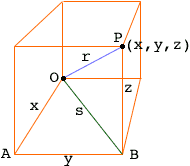Quandaries and Queries Name: jaidev Who is asking: Student Question: Is there any general equation for a sphere? Hi Jaidev, I expect you know that the equation of the circle of radius r, centered at the origin, is x2 + y2 = r2 This is just an algebraic way of stating the Theorem of Pythagoras.The point (x,y) is on the circle if and only if the right triangle with legs of length |x| and |y| has hypotenuse of length r, that is x2 + y2 = r2 For a sphere you need to use Pythagoras' theorem twice. In the diagram below O is the origin and P(x,y,z) is a point in 3-space. P is on the sphere with center O and radius r if and only if the distance from O to P is r.The triangle OAB is a right triangle and hence x2 + y2 = s2. The triangle OBP is also a right triangle and hence s2 + z2 = r2. Hence the distance |OP| from O to P satisfies x2 + y2 + z2 = |OP|2 Therefore (x,y,z) is on the sphere with center (0,0,0) and radius r if and only if x2 + y2 + z2 = r2 Similarly (x,y,z) is on the sphere with center (h,j,k) and radius r if and only if (x - h)2 + (y - j)2 + (z - k)2 = r2 Penny Go to Math Central